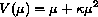Random effects Poisson regression and recurrent events data

 Speaker:  David Clayton, Institute of Public Health

With recurrent event data the total number of events for a subject will usually show dispersion between subjects which is greater than that expected from a Poisson distribution of events. One way to deal with this is to fit a Poisson regression model using the glm procedure, but to allow the scale factor to depart from 1.0. An alternative approach is to fit the Poisson model but to use a "robust" information-sandwich estimate of the variance-covariance matrix of estimates. These are provided by the author’s hglm function or by Roger Newson's more recent rglm function.

However, both of these approaches are only efficient if the variance of the event count is proportional to its expectation, and most realistic models for overdispersion do not make this prediction. It is natural to assume that overdispersion is the result of the effects of unmeasured covariates. This leads to a mixed model in which random and fixed effects are additive on the same scale. For the log link function (so that the random effects act as random multipliers or "frailties") and with Poisson errors this leads to the variance functionwhereis the frailty variance. When the frailties are distributed according to a Gamma distribution, the counts are negative binomial and the model can be fitted using nbreg. Alternatively, fixed effects can be estimated in glm using a negative binomial error structure with specified. These calculations can be alternated with estimation ofusing a program nbpar which can estimateeither using ML, maximum pseudo-likelihood, or a pseudo-REML method. Alternatively this double iteration has been implemented in the single program rpoisson. The command rpoisson is introduced to fit this model, and has the option to estimateeither by full maximum-likelihood, based on the assumption that frailties have a Gamma distribution, or by using a pseudo-likelihood forand quasi-likelihood for the regression parameters. With the maximum-likelihood option rpoisson is equivalent to the Stata command nbreg.

When the follow-up period is split into several bands either because we need to allow for time-varying covariates or for the variation of rates with time itself, subject heterogeneity introduces not only overdispersion but correlation between the counts for different time periods on the same subject. This can be dealt with in three ways:

1. Analyze the counts with hlgm or rglm, using the cluster option to ensure that the information-sandwich estimate is robust not only to misspecification of the variance function but also to correlations within clusters (here identified with subjects).
2. Analyze the marginal counts with xtpois and use the exchangeable correlation structure. This is an interface to the xtgee function. Note that approach (Clayton 1994) is also a special case of this, with independence correlation structure.
3. Model the counts at an individual level, using a random frailty effect for each record, and allowing records from the same subject to share the same frailty parameter. This leads to a multivariate extension of the variance-mean relationship which includes covariances (Clayton 1994). The model can also be fitted using rpoisson with a cluster option, which ensures that the same frailty is shared between all units within the same cluster.

Note that the first two approaches are again only efficient if the variance is proportional to the mean. rpoisson on the other hand uses variancee–covariance structures suggested by additivity of fixed and random effects on the log scale; it too allows robust estimation of standard errors using the information sandwich.

These techniques are compared using the epilepsy data (Thall and Vail 1990).

References

Clayton, D. 1994.
Some approaches to the analysis of recurrent event data. Statistical Methods in Medical Research 3: 244–262.
Thall, P. F. and S. C. Vail. 1990.
Some covariance models for longitudinal count data with overdispersion. Biometrics 46: 657–71.